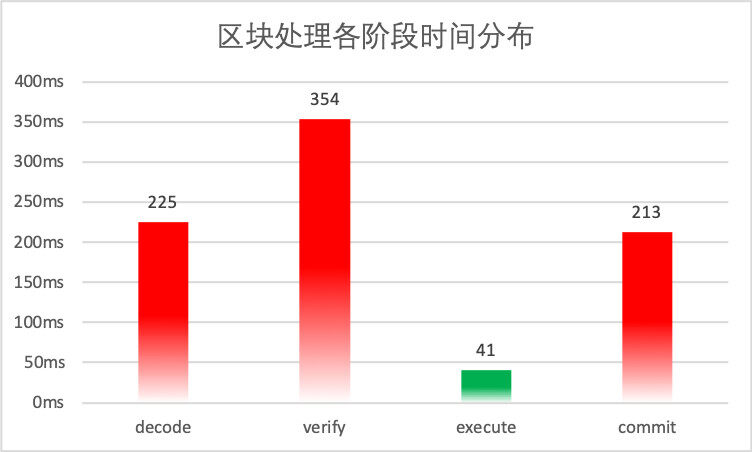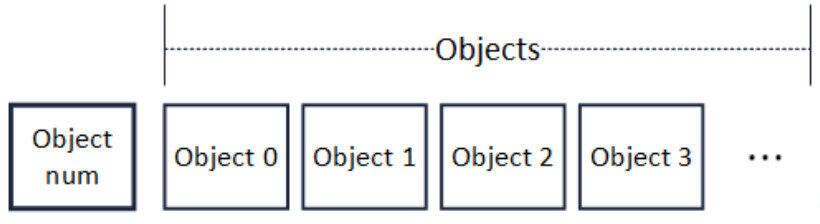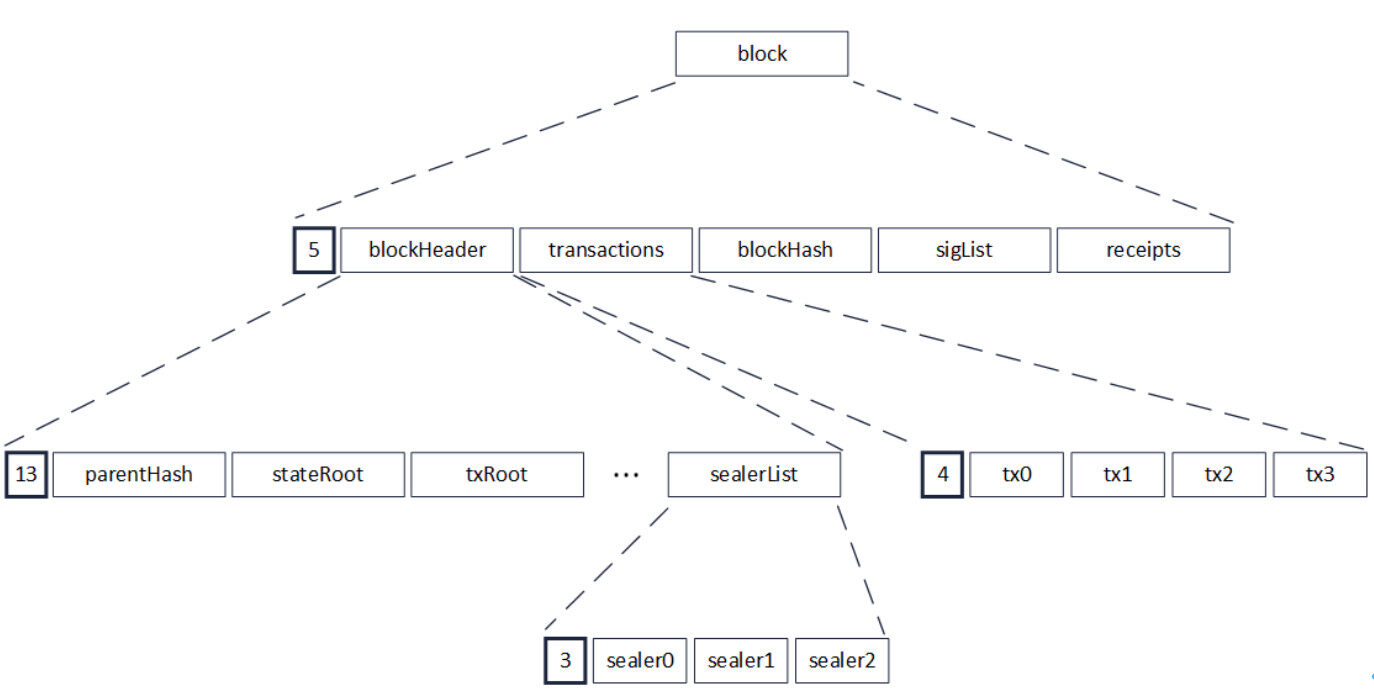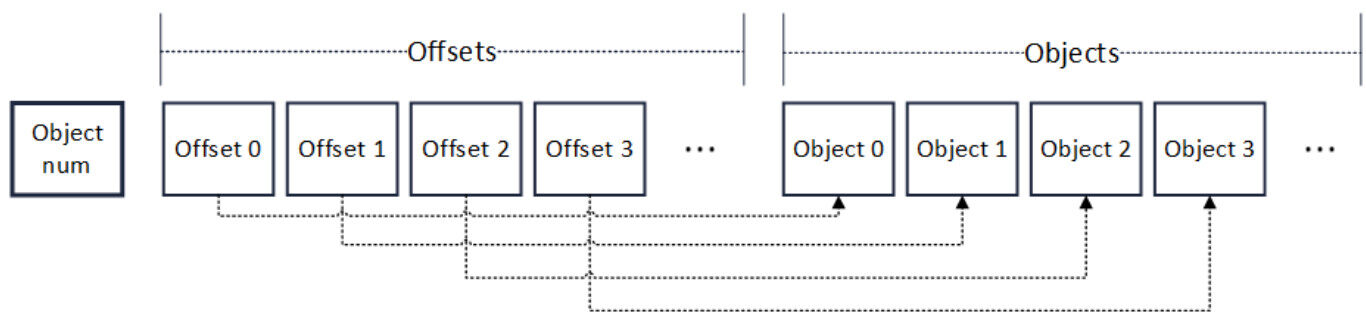# 区块链性能突破（五）：全方位的并行处理“性能”是区块链领域最受关注的话题之一，如果没有高性能表现作为支撑，就无法运行快速的、执行复杂的智能合约逻辑，从而快速完成交易事务。本系列文章将以 FISCO BCOS 开源社区对区块链性能提升的研究成果为例，阐述区块链性能优化之路。

## 背 景

PTE（Parallel Transaction Executor，一种基于 DAG 模型的并行交易执行器）的引入，使 FISCO BCOS 具备了并行执行交易的能力，显著提升了节点交易处理的效率。对这个阶段性结果，我们并不满足，继续深入挖掘发现，FISCO BCOS 的整体 TPS 仍有较大提升空间。

## 数据分析## 根因拆解

### 1. 串行的区块解码

!RLP 通过一种长度前缀与递归结合的方式，理论上可编码任意个数的对象。下图是一个区块的 RLP 编码，在对区块进行编码时，先递归至最底层，对多个 sealer 进行编码，多个 sealer 被编码并加上长度前缀后，编码成为一串 RLP 编码（sealerList），此编码又作为一个对象，被编入上层的一串 RLP 编码（blockHeader）中。此后层层递归，最后编码成为区块的 RLP 编码。由于 RLP 编码是递归的，在编码前，无法获知编码后的长度。### 2. 交易验签 & 数据落盘开销大

// 交易验签for(int i = 0; i < transactions.size(); ++i){  tx = transactions[i];  v, r, s = tx.getSignature();  publicKey = recover(v, r, s);  // 从 (v, r, s) 中复原出发送者公钥  ...}

// 数据落盘for(int i = 0; i < datas.size(); ++i){  data = datas[i];  jsonStr = jsonEncode(data);  // 将数据编码为 JSON 字符串进行存储  db.commit(jsonStr);  ...}

## 优化实践

### 1. 区块解码并行化Rlps = RLP();  // Output，初始时为空void encode(objs)  //Input: objs = 待编码对象的数组{  offset = 0;  codes = [];    objNum = objs.size()  Rlps.push(objNum)  if objNum > 1  {    for obj in objs    {      rlp = encode(obj);  // 递归调用编码方法      Rlps.push(offset);      offset += rlp.size();      codes.add(rlp);  // 缓存递归编码的结果    }    for x in codes    {      Rlps.push(x);    }  }  else  {    rlp = encode(objs);    Rlps.push(rlp);  }}

Objs decode(RLP Rlps){  objNum = Rlps.objNum；  // 获取对象个数  outputs = []  // 输出的对象数组  if objNum > 1  {    parallel for i = 0 to objNum    {      offset = Rlps.offsets[i];      code = Rlps.objs[offset];      x = decode(code);      outputs.add(x);   // 有序插入 outputs    }  }  else  {    outputs.add(decode(Rlps.objs));  }  return outputs;} 

### 2. 交易验签 & 数据落盘并行化

// 并行交易验签tbb::parallel_for(tbb::blocked_range<size_t>(0, transactions.size()), & {  for(int i = _range.begin(); i != _range.end(); ++i)  {    tx = transactions[i];    v, r, s = tx.getSignature();    publicKey = recover(v, r, s);  // 从 (v, r, s) 中复原出发送者公钥    ...  }});

// 并行数据落盘tbb::parallel_for(tbb::blocked_range<size_t>(0, transactions.size()), & {  for(int i = _range.begin(); i != _range.end(); ++i)  {    data = datas[i];    jsonStr = jsonEncode(data);  // 将数据编码为 JSON 字符串进行存储    db.commit(jsonStr);    ...  }});